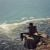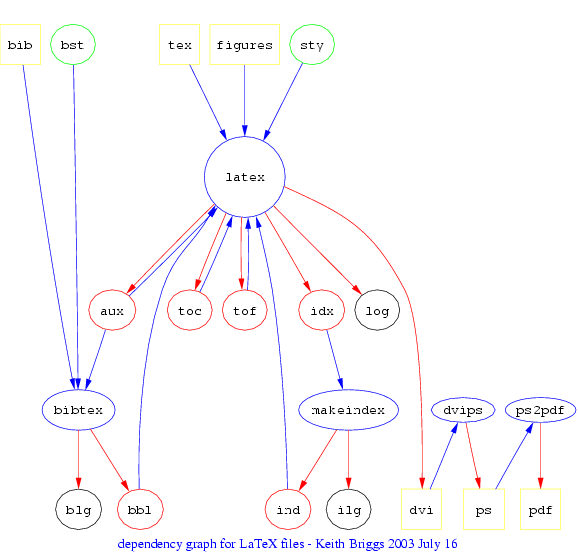# Keith Briggs (mathematician)

Updated on
Covid-19
 Name  Keith Briggs Role  Mathematician## Keith briggs thunderbolt vs grimsby

Keith Briggs is a mathematician notable for several world-record achievements in the field of computational mathematics:

## Contents

• The most accurate calculation of the Feigenbaum constants, which was published in A precise calculation of the Feigenbaum constants, Mathematics of Computation 57, 435-439.
• The worst known badly approximable irrational pair (Some explicit badly approximable pairs, Journal of Number Theory, 103, 71).
• The simplest known universal differential equation (Another universal differential equation).
• The largest number of contributions in the last 5 years to Sloane's On-Line Encyclopedia of Integer Sequences (search for briggs in OEIS). Many of these have involved major computations, such as the number of unlabelled graphs on up to 140 nodes.
• The computation of the longest sequences of colossally abundant and superabundant numbers, and their application to a test of the Riemann Hypothesis (Experimental Mathematics 15, 251-6).
• An article about him was in i-squared Magazine, Issue 6 (Winter 2008/9).

He also studies the etymology of place-names.

Topics